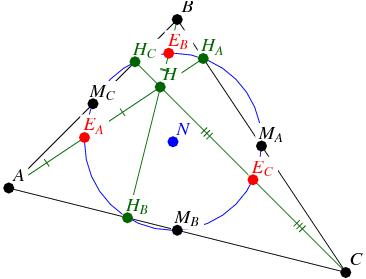FreqOn: ?→A: ?→B: ?→C: ?→D: ?→X: ?→Y:

X , Y ; . 5 DT: n(C + X◢ n(D + Y◢

n (A + X◢ n(B + Y◢ n(A + C◢ n(B + D◢

Pol( C-X , D-Y: sin( Y )(B - D) - cos( Y )(C - A→X:

C + X cos( Y◢ D + X sin( Y◢

Pol( A - maxX , B - maxY: sin( Y )(D - B) - cos( Y )(A - C→X:

A + X cos( Y◢ B + X sin( Y◢

Pol( A-C, B-D: sin( Y )(maxY - B) - cos( Y )(A - maxX→X:

A + X cos( Y◢ B + X sin( Y◢ Y→M: Pol( A - maxX , B - maxY:

( sin( M )(maxY - D) - cos( M )(C - maxX) )÷sin( Y-M→X:

C + X sin( Y→M: D - X cos( Y→Y:

n(A + M◢ n(B + Y◢ n (C + M◢

n(D + Y◢ n(maxX + M◢ n(maxY + Y

EXE (顯示6) EXE (顯示 9，即MB=(6, 9))

EXE (顯示-3) EXE (顯示 9，即MC=(-3, 9))

EXE (顯示0) EXE (顯示 0，即HA=(0, 0))

EXE (顯示6.46154) EXE (顯示 8.30769，即HB=(6.46154, 8.30769))

EXE (顯示 - 4.2) EXE (顯示 5.4，即HC=(- 4.2, 5.4))

EXE (顯示0) EXE (顯示 11，即EA=(0, 11))

EXE (顯示 - 3) EXE (顯示 2，即EB=(- 3, 2))

EXE (顯示6) EXE (顯示 2，即EC=(6, 2))

http://en.wikipedia.org/wiki/Nine-point_circle

http://www.gogeometry.com/center/nine_point_center_euler.html